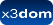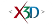## Class: IndexedFaceSet

X3D: 3.3
Component: Geometry3D
Status:
experimental

➨ Fields
➨ Properties

### HTML Encoding and Default Values⤴

``` <IndexedFaceSet attrib='x3dom.nodeTypes.X3DVertexAttributeNode' ccw='true' color='x3dom.nodeTypes.X3DColorNode' colorIndex='[]' colorPerVertex='true' convex='true' coord='x3dom.nodeTypes.X3DCoordinateNode' coordIndex='[]' creaseAngle='0' lit='true' metadata='X3DMetadataObject' normal='x3dom.nodeTypes.Normal' normalIndex='[]' normalPerVertex='true' normalUpdateMode=''fast'' solid='true' texCoord='x3dom.nodeTypes.X3DTextureCoordinateNode' texCoordIndex='[]' useGeoCache='true' ></IndexedFaceSet> ```

### Constructor⤴

#### IndexedFaceSet(ctx)⤴

Constructor for IndexedFaceSet

##### Parameters:
Name Type Argument Default Description
`ctx` Object <optional>
null context object, containing initial settings like namespace The IndexedFaceSet node represents a 3D shape formed by constructing faces (polygons) from vertices listed in the coord field.

### Fields⤴

These are the X3D / X3DOM fields of this node. Values should usually be received / set as strings via DOM functions (i.e., using `setAttribute("myFieldName", "myFieldValue")` and `getAttribute("myFieldName")`).
Name Type Default Value Range Inheritance Standard Description
attrib MFNode X3DVertexAttributeNode X3DComposedGeometryNodeIf the attrib field is not empty it shall contain a list of per-vertex attribute information for programmable shaders.
ccw SFBool true X3DGeometryNodeThe ccw field defines the ordering of the vertex coordinates of the geometry with respect to user-given or automatically generated normal vectors used in the lighting model equations.
color SFNode X3DColorNode X3DComposedGeometryNodeIf the color field is NULL, the geometry shall be rendered normally using the Material and texture defined in the Appearance node.
colorIndex MFInt32 []The index data for the color data.
colorPerVertex SFBool true X3DComposedGeometryNodeIf colorPerVertex is FALSE, colours are applied to each face. If colorPerVertex is true, colours are applied to each vertex.
convex SFBool trueThe convex field indicates whether all polygons in the shape are convex (TRUE). A polygon is convex if it is planar, does not intersect itself, and all of the interior angles at its vertices are less than 180 degrees.
coord SFNode X3DCoordinateNode X3DComposedGeometryNodeContains a Coordinate node.
coordIndex MFInt32 []The index data for the coord data.
creaseAngle SFFloat 0The creaseAngle field affects how default normals are generated. If the angle between the geometric normals of two adjacent faces is less than the crease angle, normals shall be calculated so that the faces are shaded smoothly across the edge; otherwise, normals shall be calculated so that a lighting discontinuity across the edge is produced. Crease angles shall be greater than or equal to 0.0 angle base units.
lit SFBool true X3DGeometryNodeSpecifies whether this geometry should be rendered with or without lighting.
metadata SFNode X3DMetadataObject X3DNodeField to add metadata information
normal SFNode Normal X3DComposedGeometryNodeIf the normal field is not NULL, it shall contain a Normal node whose normals are applied to the vertices or faces of the X3DComposedGeometryNode in a manner exactly equivalent to that described above for applying colours to vertices/faces (where normalPerVertex corresponds to colorPerVertex and normalIndex corresponds to colorIndex). If the normal field is NULL, the browser shall automatically generate normals in accordance with the node's definition. If the node does not define a behaviour, the default is to generate an averaged normal for all faces that share that vertex.
normalIndex MFInt32 []The index data for the normal data.
normalPerVertex SFBool true X3DComposedGeometryNodeIf normalPerVertex is FALSE, normals are applied to each face. If normalPerVertex is true, normals are applied to each vertex.
normalUpdateMode SFString 'fast' X3DComposedGeometryNodesolid SFBool true X3DGeometryNodeSpecifies whether backface-culling is used. If solid is TRUE only front-faces are drawn.
texCoord SFNode X3DTextureCoordinateNode X3DComposedGeometryNodeIf the texCoord field is not NULL, it shall contain a TextureCoordinate node.
texCoordIndex MFInt32 []The index data for the texcoord data.
useGeoCache SFBool true X3DGeometryNodeMost geo primitives use geo cache and others might later on, but one should be able to disable cache per geometry node. Defaults to global useGeoCache setting parameter.